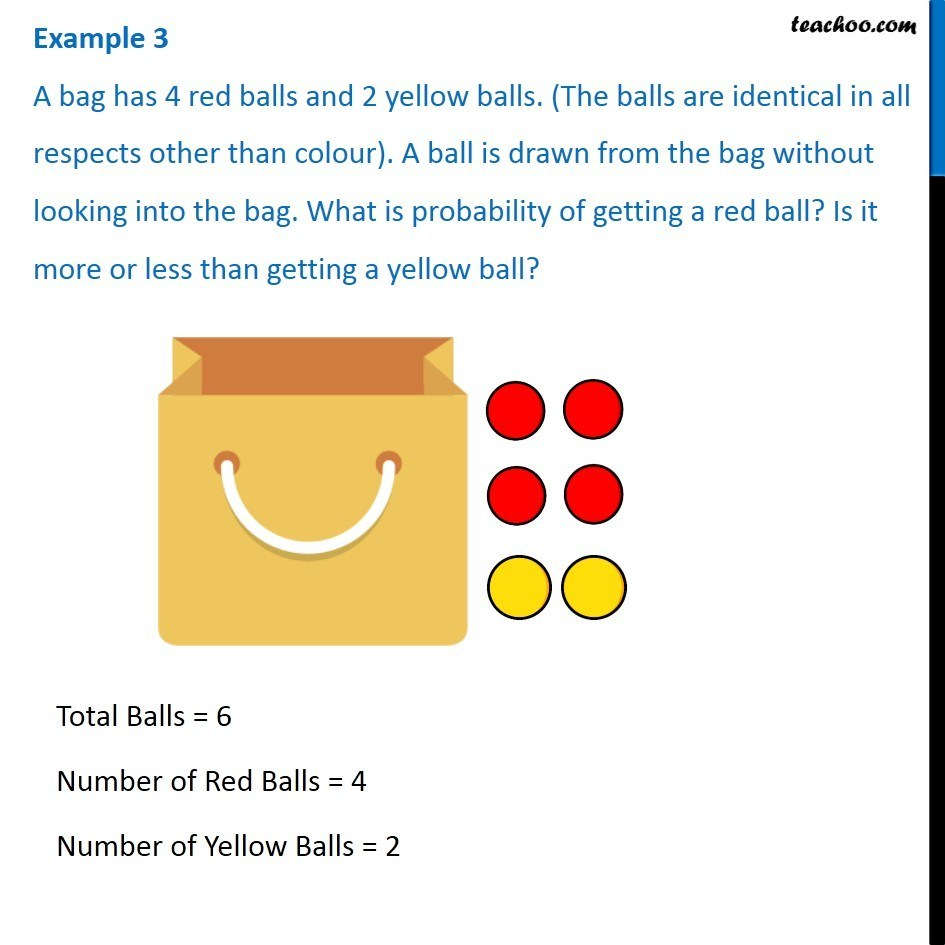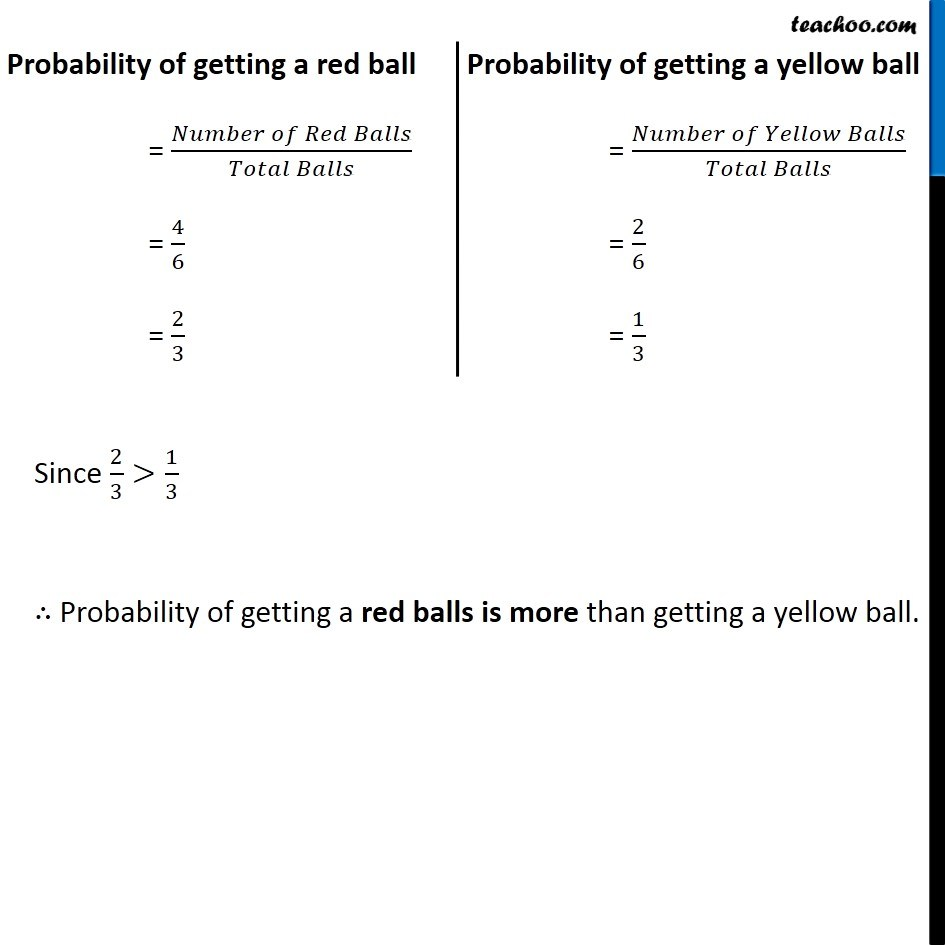Probability

Chapter 5 Class 8 Data Handling
Concept wiseIntroducing your new favourite teacher - Teachoo Black, at only ₹83 per month

### Transcript

Example 3 A bag has 4 red balls and 2 yellow balls. (The balls are identical in all respects other than colour). A ball is drawn from the bag without looking into the bag. What is probability of getting a red ball? Is it more or less than getting a yellow ball? Total Balls = 6 Number of Red Balls = 4 Number of Yellow Balls = 2 Probability of getting a red ball = (𝑁𝑢𝑚𝑏𝑒𝑟 𝑜𝑓 𝑅𝑒𝑑 𝐵𝑎𝑙𝑙𝑠)/(𝑇𝑜𝑡𝑎𝑙 𝐵𝑎𝑙𝑙𝑠) = 4/6 = 2/3 Probability of getting a yellow ball = (𝑁𝑢𝑚𝑏𝑒𝑟 𝑜𝑓 𝑌𝑒𝑙𝑙𝑜𝑤 𝐵𝑎𝑙𝑙𝑠)/(𝑇𝑜𝑡𝑎𝑙 𝐵𝑎𝑙𝑙𝑠) = 2/6 = 1/3 Since 2/3>1/3 ∴ Probability of getting a red balls is more than getting a yellow ball.